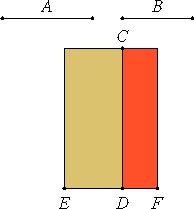# Proposition 23

A straight line commensurable with a medial straight line is medial.

Let A be medial, and let B be commensurable with A.

I say that B is also medial.

X.22

Set out a rational straight line CD. Apply the rectangular area CE to CD equal to the square on A, producing ED as breadth. Then ED is rational and incommensurable in length with CD. And apply the rectangular area CF to CD equal to the square on B, producing DF as breadth.Since A is commensurable with B, therefore the square on A is also commensurable with the square on B. But EC equals the square on A, and CF equals the square on B, therefore EC is commensurable with CF.

And EC is to CF as ED is to DF, therefore ED is commensurable in length with DF.

But ED is rational and incommensurable in length with DC, therefore DF is also rational and incommensurable in length with DC.

Therefore CD and DF are rational and commensurable in square only.

X.21

But the straight line is medial on which the square equals the rectangle contained by rational straight lines commensurable in square only, therefore the side of the square equals the rectangle CD by DF is medial.

And B is the side of the square equal the rectangle CD by DF, therefore B is medial.

Therefore, a straight line commensurable with a medial straight line is medial.

Q.E.D.

# Corollary

From this it is clear that an area commensurable with a medial area is medial.

# Note

X.18,Lemma

And in the same way as was explained in the case of rationals it follows regards medials, that a straight line commensurable in length with a medial straight line is called medial and commensurable with it not only in length but in square also, since, in general, straight lines commensurable in length are always commensurable in square also.

But, if any straight line is commensurable in square with a medial straight line, then if it is also commensurable in length with it, the straight lines are called, in this case too, medial and commensurable in length and in square, but, if in square only, they are called medial straight lines commensurable in square only.

## Guide

We can interpret this proposition numerically. We defined a medial number in the Guide to X.21 as being a number whose square is irrational but whose fourth power is a rational number. Then this proposition says that the quotient of a medial number and a rational number is a medial number. Likewise, the product of a medial number and a rational number is a medial number.

#### Use of this proposition

The proposition is used in X.67 and X.104, the corollary in X.33 and many others, and the note in X.27 and a few others.